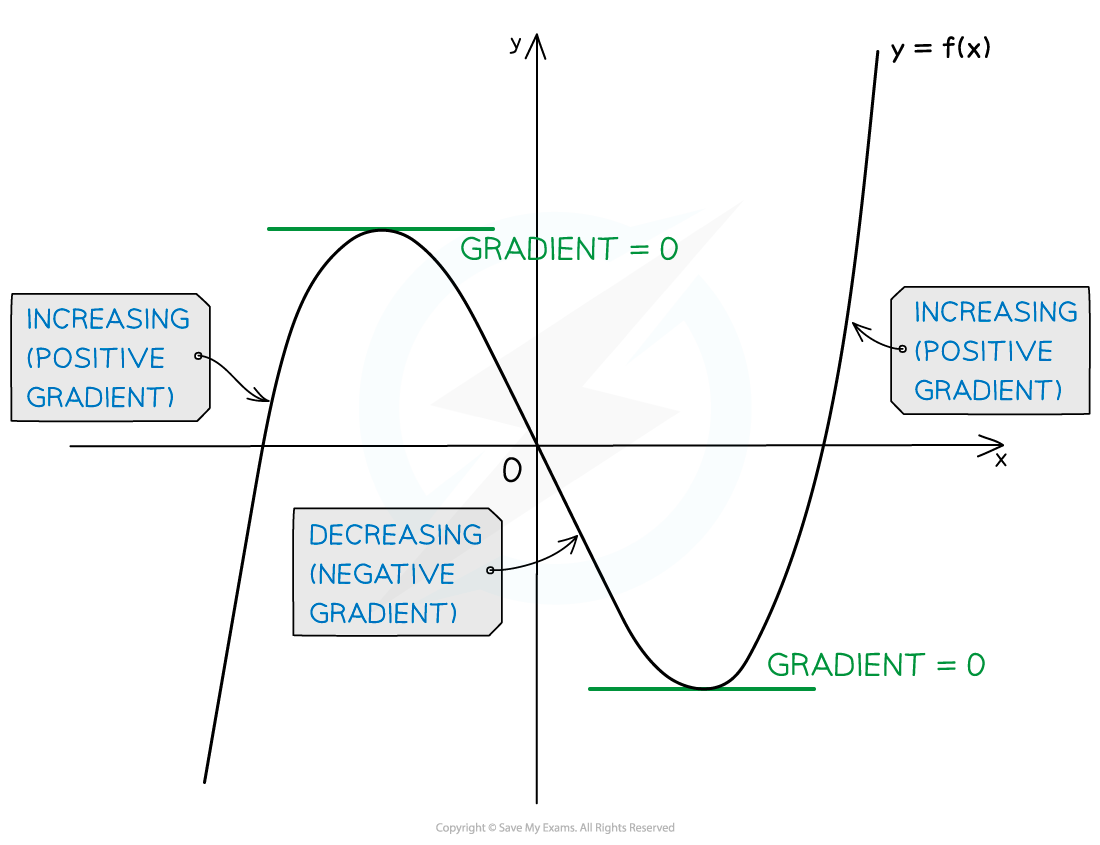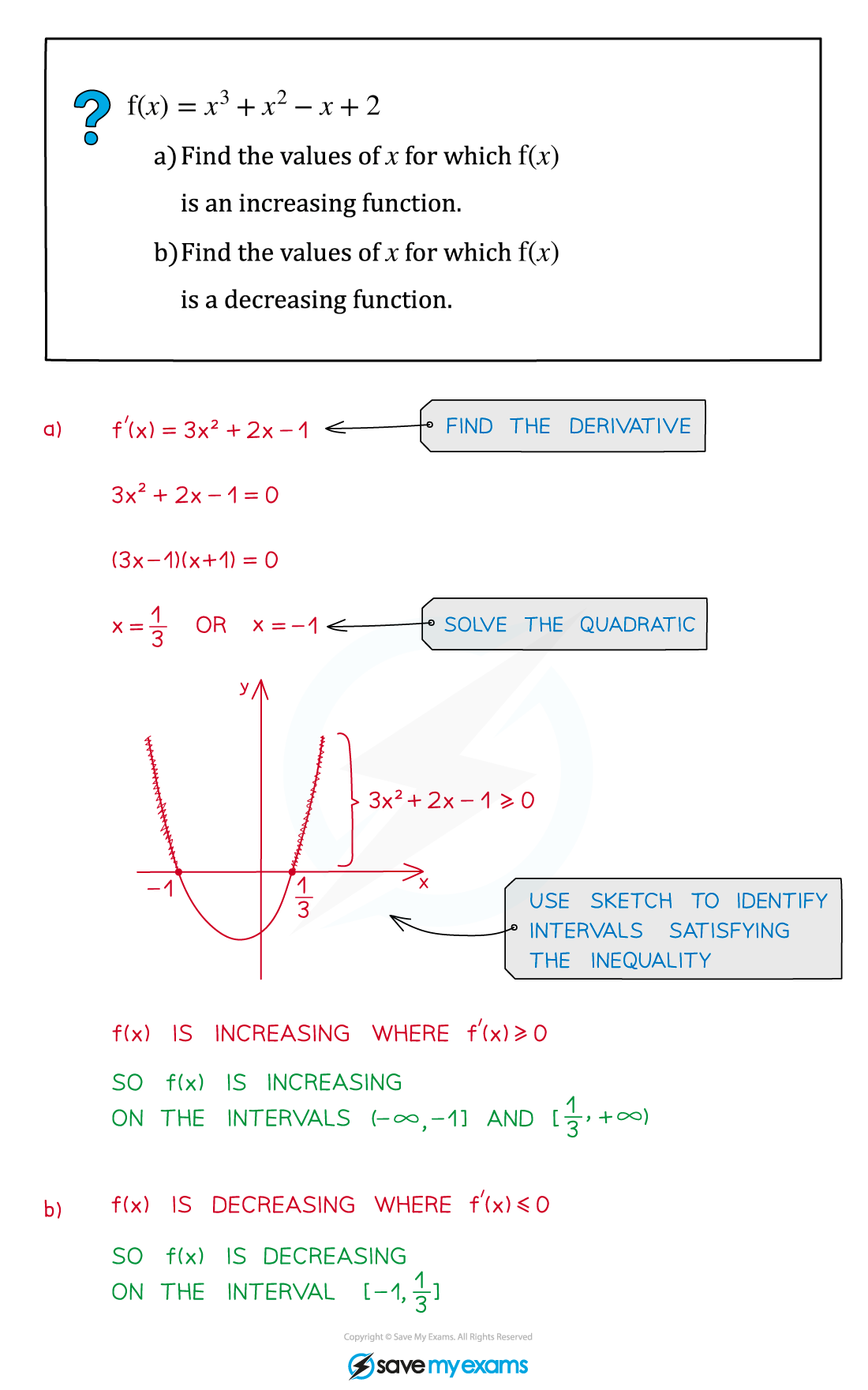# AQA A Level Maths: Pure复习笔记7.2.2 Increasing & Decreasing Functions

### Increasing & Decreasing Functions

#### What are increasing and decreasing functions?

• A function f(x) is increasing on an interval [a, b] if f'(x) ≥ 0 for all values of x such that a< x < b.
• If f'(x) > 0 for all x values in the interval then the function is said to be strictly increasing
• In most cases, on an increasing interval the graph of a function goes up as x increases
• A function f(x) is decreasing on an interval [a, b] if f'(x) ≤ 0 for all values of x such that a < x < b
• If f'(x) < 0 for all x values in the interval then the function is said to be strictly decreasing
• In most cases, on a decreasing interval the graph of a function goes down as x increases• To identify the intervals on which a function is increasing or decreasing you need to:
1. Find the derivative f'(x)
2. Solve the inequalities f'(x) ≥ 0 (for increasing intervals) and/or f'(x) ≤ 0 (for decreasing intervals)

#### Exam Tip

• On an exam, if you need to show a function is increasing or decreasing you can use either strict (<, >) or non-strict (≤, ≥) inequalities

#### Worked Example